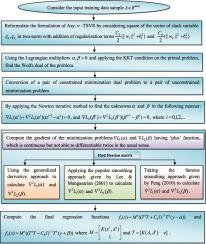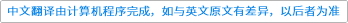Applied Soft Computing ( IF 5.472 ) Pub Date : 2021-01-13 , DOI: 10.1016/j.asoc.2021.107099
Deepak Gupta; Umesh GuptaThe main objective of twin support vector regression (TSVR) is to find the optimum regression function based on the $\epsilon$-insensitive up- and down-bound with equal influences on the regression function where all the data points have a different location above the up-bound points and below the down-bound points. However, the effects of all data points must be distinct based on their distribution in the regression function. Recently, asymmetric v-twin support vector regression (Asy-v-TSVR) is encouraged on the same subject but still, the present matrices in the mathematical formulation have faced the problem of semi-definite. In order to handle this problem effectively, a new regressor model named as robust asymmetric Lagrangian v-twin support vector regression using pinball loss function (URALTSVR) proposes as a pair of the unconstrained minimization problem to handle not only the noise sensitivity and instability of re-sampling but also consist positive definite matrices. Here, we suggest the proposed model URALTSVR in such a way where the pinball loss function is playing a vital role to control the fitting error inside the asymmetric tube. One of the advantages is that unlike TSVR and Asy-v-TSVR, it considers the concept of structural risk minimization principle through the inclusion of regularization term as well as change the one-norm of the vector of the slack variable by two-norm, which yields the dual problem to be strongly convex, stable and well-posed. Aforementioned, the proposed formulation has a continuous and piecewise quadratic problem that is solved by their gradients based iterative approaches. Specifically, we analyze the three implementations of URALTSVR with the baselines approaches support vector regression (SVR), TSVR and Asy-v-TSVR, which discard the dependencies to solve a pair of quadratic programming problem (QPP) for obtaining the unique global solution. Overall, SRALTSVR1 based on smooth approximation function performs outstanding for artificial and real-world datasets.down
wechat
bug